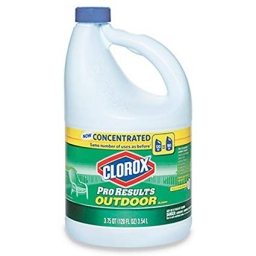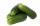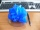# Home cleaning

Mr. Smith is cleaning up a big mess at home. In the closet, he finds a solution that is 5% bleach, and another stronger solution that is 20% bleach. For this particular job, he needs 600mL of 15% bleach. How much of each type (to the nearest mL) should he mix together to do this cleaning job?

a =  200
b =  400

### Step-by-step explanation:

a+b = 600
0.05a + 0.20b = 0.15 • 600

a+b = 600
0.05•a + 0.20•b = 0.15 • 600

a+b = 600
0.05a+0.2b = 90

a = 200
b = 400

Our linear equations calculator calculates it.Did you find an error or inaccuracy? Feel free to write us. Thank you!Tips to related online calculators
Do you have a linear equation or system of equations and looking for its solution? Or do you have a quadratic equation?
Tip: Our volume units converter will help you with the conversion of volume units.

## Related math problems and questions:

• Solutions, mixturesWe have 2 liters of 20% solution available. How much 70% solution do we need to add to it to get a 30% solution?
• SolutionsHow much 60% solution and how much 35% solution is needed to create 100 l of 40% solution?
• GramsHow many grams of 5% solution do we need to add to 100g of 50% solution to get a 20% solution?
• PharmacyAt the pharmacy are in one container 20% solution in the second 50% solution of disinfectant. They need to prepare 4 L of 48-percent solution. What amount of solution from each container is needed to mix?
• Disinfecting solutionHow much distilled water is necessary to pour into 500 ml of 33% hydrogen peroxide solution to obtain 3% disinfecting solution?
• PeroxideHow many ml should we pour 30% of peroxide (H2O2) into 100ml H2O to give a 20% solution?
• SolutionWhat amount of 80% solution and what amount of water is needed to mix in order to obtain two liters of 60% solution of this mixture?
• CuSO4 mixtureHow many grams of solid CuSO4 we have to add to 450g of 15% CuSO4 solution to produce a 25% solution?
• Water solutionHow many grams of salt should we dissolve in 400 g of water to get a 20% solution?
• Alcohol 2Two types of alcohol one 63% and second 75% give 20 liters of 69% alcohol. How many liters of each type are in the mixture?
• Energy savingThey were released three different, independent inventions saving 20%, 24% and 15% energy. Some considered that while the use of these inventions, the total savings will be 20% + 24% + 15% = 59%. Is this true? How much percent of energy will save all thre
• Acid solutionBy adding 250 grams of a 96% sulfuric acid solution to its 3% solution, we changed its initial concentration to 25%. How many grams of 3% of the acid was used for dilution?
• FertilizerMr. Gherkin use for fertilization 7% solution of fertilizer. 17 liters of it still left. How much water must be added to the solution to make only 3% solution?
• Alcohol solutionsWe have to produce 2 liters of 60% alcohol from 55% and 80%. How many of which ones will we use in the solution?
• Copper sulphateHow much g of water do we have to add to 240 g of an 84% CuSO4 solution to produce a 60% solution? (Express the mass of crystalline CuSO4 in the original solution and in the resulting solution and compare them. )
• Cinema ticketsCinema sold 180 tickets this Thursday, which is 20%. Monday 14%, Tuesday 6%, Wednesday 9%, Friday 24%, Saturday 12%, and Sunday 15%. How many tickets were sold per week?
• Gasoline-oil ratioThe manufacturer of a scooter engine recommends a gasoline-oil fuel mixture ratio of 15 to 1. In a particular garage, we can buy pure gasoline and a gasoline-oil mixture, 75% gasoline. How much gasoline and gasoline-oil mix do we need to make 8.0 L of fue